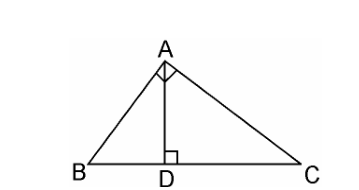Guru

# D is a point on the side BC of a triangle ABC such that ∠ADC = ∠BAC. Show that CA2 = CB.CD Q.13

• 0

How to solve the problem of Triangles of exercise 6.3 of class 10th, I don’t know how to solve this question please guide me the best way to solve this problem. D is a point on the side BC of a triangle ABC such that ∠ADC = ∠BAC. Show that CA2 = CB.CD

Share

1. Given, D is a point on the side BC of a triangle ABC such that ∠ADC = ∠BAC.∠ACD = ∠BCA (Common angles)

∴ ΔADC ~ ΔBAC (AA similarity criterion)

We know that corresponding sides of similar triangles are in proportion.

∴ CA/CB = CD/CA

⇒ CA2 = CB.CD.

Hence, proved.

• 0